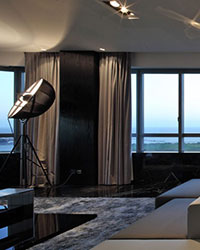# Browse By LIVING ROOMLIVING ROOM Description:

The living room is the most important room in the house because it's usually the place the family spends the most time. There are many different uses for the room, so it's important to make sure you have the appropriate lighting.

There's actually a proven formula for calculating the proper amount of general light (usually expressed as the number of watts) you should have in each room or area of your home . . . and it's not difficult, just some basic arithmetic.

Multiply the length times the width of the room. Then, multiply that number times 1.5. That gives you the amount of wattage you need to light the room properly for general illumination. Example: A room is 12 ft. x 16 ft. (12 x 16 = 192). Then multiply 192 x 1.5 = 288 watts. That means an 8-light chandelier using 40-watt bulbs would give 320 watts, which is even more light than needed.

For specific task lighting in areas where stronger light is needed, multiply the area's square footage by 2.5 rather than 1.5 to find the needed wattage. A desk area where schoolwork is done is an example of a task area in your home. Instructions provided by Kichler Lighting.